# KR20160147435A - Method of estimating a yield of an integrated circuit and method of optimizing a design for an integrated circuit - Google Patents

Method of estimating a yield of an integrated circuit and method of optimizing a design for an integrated circuit Download PDF

## Info

Publication number
KR20160147435A
KR20160147435A KR1020150084181A KR20150084181A KR20160147435A KR 20160147435 A KR20160147435 A KR 20160147435A KR 1020150084181 A KR1020150084181 A KR 1020150084181A KR 20150084181 A KR20150084181 A KR 20150084181A KR 20160147435 A KR20160147435 A KR 20160147435A
Authority
KR
South Korea
Prior art keywords
critical
sigma level
integrated circuit
threshold
yield
Prior art date
Application number
KR1020150084181A
Other languages
Korean (ko)
Inventor
김문수
임경환
배철준
Original Assignee
삼성전자주식회사
Priority date (The priority date is an assumption and is not a legal conclusion. Google has not performed a legal analysis and makes no representation as to the accuracy of the date listed.)
Filing date
Publication date
Application filed by 삼성전자주식회사 filed Critical 삼성전자주식회사
Priority to KR1020150084181A priority Critical patent/KR20160147435A/en
Publication of KR20160147435A publication Critical patent/KR20160147435A/en

• 238000004458 analytical methods Methods 0 abstract 1
• 230000003068 static Effects 0 abstract 1

## Images

•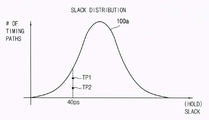•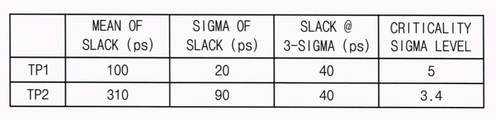•••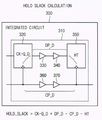•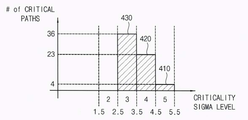•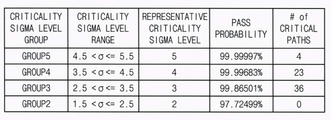•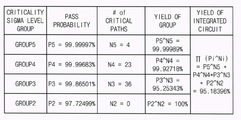•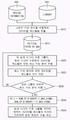•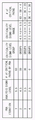•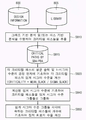•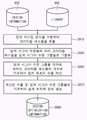•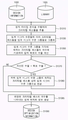•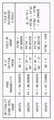••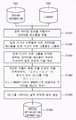••## Classifications

• GPHYSICS
• G06COMPUTING; CALCULATING; COUNTING
• G06FELECTRIC DIGITAL DATA PROCESSING
• G06F17/00Digital computing or data processing equipment or methods, specially adapted for specific functions
• G06F17/50Computer-aided design
• G06F17/5009Computer-aided design using simulation
• G06F17/5022Logic simulation, e.g. for logic circuit operation
• G06F17/5031Timing analysis
• GPHYSICS
• G06COMPUTING; CALCULATING; COUNTING
• G06FELECTRIC DIGITAL DATA PROCESSING
• G06F17/00Digital computing or data processing equipment or methods, specially adapted for specific functions
• G06F17/50Computer-aided design
• G06F17/5045Circuit design
• G06F17/505Logic synthesis, e.g. technology mapping, optimisation
• GPHYSICS
• G06COMPUTING; CALCULATING; COUNTING
• G06FELECTRIC DIGITAL DATA PROCESSING
• G06F17/00Digital computing or data processing equipment or methods, specially adapted for specific functions
• G06F17/50Computer-aided design
• G06F17/5045Circuit design
• GPHYSICS
• G06COMPUTING; CALCULATING; COUNTING
• G06FELECTRIC DIGITAL DATA PROCESSING
• G06F17/00Digital computing or data processing equipment or methods, specially adapted for specific functions
• G06F17/50Computer-aided design
• G06F17/5068Physical circuit design, e.g. layout for integrated circuits or printed circuit boards
• GPHYSICS
• G06COMPUTING; CALCULATING; COUNTING
• G06FELECTRIC DIGITAL DATA PROCESSING
• G06F17/00Digital computing or data processing equipment or methods, specially adapted for specific functions
• G06F17/50Computer-aided design
• G06F17/5068Physical circuit design, e.g. layout for integrated circuits or printed circuit boards
• G06F17/5081Layout analysis, e.g. layout verification, design rule check
• GPHYSICS
• G06COMPUTING; CALCULATING; COUNTING
• G06FELECTRIC DIGITAL DATA PROCESSING
• G06F2217/00Indexing scheme relating to computer aided design [CAD]
• G06F2217/84Timing analysis and optimization

## Abstract

In a method for predicting the yield of an integrated circuit, static timing analysis is performed on the integrated circuit to extract critical ones of the timing paths included in the integrated circuit, and the critical paths are grouped into critical sigma level groups according to the critical sigma levels . The yield of the integrated circuit is calculated based on the number of critical passes belonging to each of the critical sigma level groups. Thus, the yield of the integrated circuit can be predicted more accurately and can be calculated efficiently.

## Description

 METHOD OF ESTIMATING A YIELD OF AN INTEGRATED CIRCUIT AND METHOD OF OPTIMIZING A DESIGN FOR AN INTEGRATED CIRCUIT 

The present invention relates to semiconductor circuit design and more particularly to a method for predicting the yield of an integrated circuit and a method for optimizing the design of the integrated circuit.

In designing an integrated circuit such as a system-on-chip (SOC), a static timing analysis (STA) is performed for timing analysis of a designed integrated circuit. This static timing analysis can improve the yield of the integrated circuit by reflecting the process variation in the design stage of the integrated circuit. That is, the static timing analysis can determine the timing pass or failure of the timing path by calculating the slack of each timing path, and the designer of the integrated circuit can calculate the slack of the calculated slack The design of the integrated circuit may be modified or supplemented. However, since the slack calculated by the static timing analysis can not express the probability information on timing success or failure, there is a problem that it is difficult to predict the yield of the integrated circuit based on such slack.

It is an object of the present invention to provide a method for predicting the yield of an integrated circuit capable of efficiently predicting the yield of an integrated circuit.

It is another object of the present invention to provide a design optimization method of an integrated circuit capable of optimizing the design of an integrated circuit through prediction of the yield of the integrated circuit.

It is to be understood, however, that the present invention is not limited to the above-described embodiments and various modifications may be made without departing from the spirit and scope of the invention.

In order to accomplish the above object, in a method for predicting the yield of an integrated circuit according to embodiments of the present invention, a static timing analysis is performed on the integrated circuit so that a timing path included in the integrated circuit critical paths are extracted and critical paths are grouped into critical sigma level groups according to the critical sigma levels of the critical paths and the critical paths of the critical paths belonging to each of the critical sigma level groups The yield of the integrated circuit is calculated based on the number.

In one embodiment, each of the critical passes may have a slack of zero or less at a sigma level above the critical sigma level.

In one embodiment, the critical passes may be extracted by performing Graph Based Analysis (GBA) on the integrated circuit as the static timing analysis.

In one embodiment, a Path Based Analysis (PBA) is performed on the critical paths at the maximum critical sigma levels of the critical sigma level groups to group the critical paths into the threshold sigma level groups , The number of critical passes belonging to each of the threshold sigma level groups may be calculated based on the results of the path based analysis at the maximum threshold sigma levels of the threshold sigma level groups.

In one embodiment, the number of critical passes belonging to a first threshold sigma level group of the threshold sigma level groups is greater than the number of critical passes at the maximum threshold sigma level of the first threshold sigma level group, Can be calculated as the difference of the number of critical passes at the maximum critical sigma level of the second critical sigma level group of sigma level groups.

In one embodiment, the threshold sigma levels of the critical passes are calculated based on mean slacks and 1-sigma level process deviations of the critical passes to group the critical passes into the threshold sigma level groups, The critical paths may be grouped into the threshold sigma level groups based on the calculated threshold sigma levels.

In one embodiment, the critical sigma level of each of the critical passes may be calculated by dividing the average slack of each of the critical passes by the process deviation of the 1-sigma level of each of the critical passes.

In one embodiment, the yields of the threshold sigma level groups are calculated by multiplying the success probability of each of the threshold sigma level groups by the number of critical passes belonging to each of the threshold sigma level groups, so as to calculate the yield of the integrated circuit And the yield of the integrated circuit may be calculated by multiplying the calculated yields of the threshold sigma level groups.

According to another aspect of the present invention, there is provided a method of optimizing an integrated circuit according to embodiments of the present invention, the method comprising: performing a static timing analysis on the integrated circuit, critical paths are extracted and critical paths are grouped into critical sigma level groups according to the critical sigma levels of the critical paths and the critical paths of the critical paths belonging to each of the critical sigma level groups The yield of the integrated circuit is calculated based on the number, and design optimization information is generated based on the calculated yield of the integrated circuit and the critical sigma level groups.

In one embodiment, the design optimization information includes information indicating the number of critical passes that must be changed from a first critical sigma level group of the critical sigma level groups to a second critical sigma level group of the critical sigma level groups . &Lt; / RTI &gt;

In one embodiment, the second threshold sigma level group may have a representative threshold sigma level higher than the representative threshold sigma level of the first threshold sigma level group.

In one embodiment, the design optimization information includes information indicating the number of critical passes that should be changed from a third threshold sigma level group of the threshold sigma level groups to a fourth threshold sigma level group of the threshold sigma level groups And the fourth threshold sigma level group may have a representative threshold sigma level lower than the representative threshold sigma level of the third threshold sigma level group.

In one embodiment, the calculated yield of the integrated circuit may be compared to a target yield for the integrated circuit, and the design optimization information may be generated when the calculated yield is lower than the target yield.

In one embodiment, to generate the design optimization information, at least one of the first threshold sigma level group having the lowest representative threshold sigma level among the threshold sigma level groups, until the calculated yield reaches or exceeds the target yield rate, The critical path is changed to a second threshold sigma level group having a representative threshold sigma level higher than the lowest representative threshold sigma level, and the number of critical paths changed from the first threshold sigma level group to the second threshold sigma level group The design optimization information indicating the design optimization information may be generated.

In one embodiment, to generate the design optimization information, a set of X ijs that satisfies that the calculated yield is greater than or equal to the target yield for the integrated circuit and minimizes the expression "((ji) * X ij)" , The design optimization information indicating the set of Xij can be generated. Where i is an integer representing the representative threshold sigma level of the critical sigma level group to which the critical path belongs before optimization and j is an integer representing the representative threshold sigma level of the critical sigma level group to which the critical path belongs after the optimization , And Xij may represent the number of critical passes that are changed to the threshold sigma level group having the representative threshold sigma level of j in the threshold sigma level group having the representative threshold sigma level of i.

In one embodiment, the set of Xijs may be computed by performing integer linear programming on the equation "[Sigma ((j-i) * Xij) ".

According to another aspect of the present invention, there is provided a method of optimizing an integrated circuit according to embodiments of the present invention, the method comprising: performing a Graph Based Analysis (GBA) on the integrated circuit, a critical path among the timing paths is extracted, a path based analysis (PBA) is performed on the critical paths at the maximum critical sigma levels of the critical sigma level groups, Wherein the number of critical passes belonging to each of the threshold sigma level groups is calculated based on the results of the path based analysis at the maximum threshold sigma levels of the level groups, Wherein the yield of the integrated circuit is calculated based on the number of passes, The calculated yield is compared to the target yield for the integrated circuit, and design optimization information can be generated based on the result of the comparison of the calculated yield and the target yield.

In one embodiment, the yields of the threshold sigma level groups are calculated by multiplying the success probability of each of the threshold sigma level groups by the number of critical passes belonging to each of the threshold sigma level groups, so as to calculate the yield of the integrated circuit And the yield of the integrated circuit may be calculated by multiplying the calculated yields of the threshold sigma level groups.

In one embodiment, the design optimization information includes information indicating the number of critical passes that must be changed from a first critical sigma level group of the critical sigma level groups to a second critical sigma level group of the critical sigma level groups And the second threshold sigma level group may have a representative threshold sigma level higher than the representative threshold sigma level of the first threshold sigma level group.

In one embodiment, to generate the design optimization information, at least one of the first threshold sigma level group having the lowest representative threshold sigma level among the threshold sigma level groups, until the calculated yield reaches or exceeds the target yield rate, The critical path is changed to a second threshold sigma level group having a representative threshold sigma level higher than the lowest representative threshold sigma level and the number of critical paths changed from the first threshold sigma level group to the second threshold sigma level group The design optimization information indicating the design optimization information may be generated.

The method of predicting the yield of the integrated circuit and the method of optimizing the design of the integrated circuit according to the embodiments of the present invention may be applied to the criticality sigma level of the critical paths extracted by the static timing analysis (STA) The critical paths may be grouped into critical sigma level groups and the yield of the integrated circuit may be efficiently calculated based on the critical sigma level groups.

In addition, the method of predicting the yield of the integrated circuit and the method of optimizing the design of the integrated circuit according to the embodiments of the present invention can efficiently optimize the design of the integrated circuit based on the efficiency of the efficiently calculated integrated circuit.

However, the effects of the present invention are not limited to the above-mentioned effects, and may be variously expanded without departing from the spirit and scope of the present invention.

1A is a diagram showing an example of a slack distribution of an integrated circuit.
1B is a diagram showing examples of threshold sigma levels of first and second timing passes in the slack distribution of FIG. 1A.
1C is a diagram illustrating an example of a critical sigma level distribution of an integrated circuit having the slack distribution of FIG. 1A.
2 is a flow chart illustrating a method for predicting yield of an integrated circuit according to embodiments of the present invention.
3 is a diagram for explaining an example of calculating a hold slack of a timing path included in an integrated circuit.
Figures 4A and 4B are diagrams illustrating an example in which critical passes are grouped into critical sigma level groups according to threshold sigma levels.
5 is a diagram illustrating an example of calculating the yield of an integrated circuit based on the number of critical passes belonging to each of the critical sigma level groups.
6 is a flowchart illustrating a method of predicting yield of an integrated circuit according to an embodiment of the present invention.
FIG. 7 is a diagram for explaining an example of grouping critical paths by performing path-based analysis in the yield prediction method of FIG.
8 is a flowchart illustrating a method of predicting yield of an integrated circuit according to another embodiment of the present invention.
9 is a flowchart illustrating a method for optimizing a design of an integrated circuit according to embodiments of the present invention.
10 is a flowchart illustrating a design optimization method of an integrated circuit according to an embodiment of the present invention.
11 is a diagram for explaining an example in which the yield of an integrated circuit is improved by the design optimization method of FIG.
12 is a diagram for explaining an example in which the critical sigma level distribution of an integrated circuit is improved by the design optimization method of FIG.
13 is a flowchart illustrating a method of optimizing a design of an integrated circuit according to another embodiment of the present invention.
14 is a diagram for explaining an example in which the critical sigma level distribution of an integrated circuit is improved by the design optimization method of FIG.
15 is a diagram illustrating a computing system that performs a method of predicting the yield of an integrated circuit according to embodiments of the present invention.

For the embodiments of the invention disclosed herein, specific structural and functional descriptions are set forth for the purpose of describing an embodiment of the invention only, and it is to be understood that the embodiments of the invention may be practiced in various forms, The present invention should not be construed as limited to the embodiments described in Figs.

The present invention is capable of various modifications and various forms, and specific embodiments are illustrated in the drawings and described in detail in the text. It is to be understood, however, that the invention is not intended to be limited to the particular forms disclosed, but on the contrary, is intended to cover all modifications, equivalents, and alternatives falling within the spirit and scope of the invention.

The terms first, second, etc. may be used to describe various components, but the components should not be limited by the terms. The terms may be used for the purpose of distinguishing one component from another. For example, without departing from the scope of the present invention, the first component may be referred to as a second component, and similarly, the second component may also be referred to as a first component.

It is to be understood that when an element is referred to as being "connected" or "connected" to another element, it may be directly connected or connected to the other element, . On the other hand, when an element is referred to as being "directly connected" or "directly connected" to another element, it should be understood that there are no other elements in between. Other expressions that describe the relationship between components, such as "between" and "between" or "neighboring to" and "directly adjacent to" should be interpreted as well.

The terminology used in this application is used only to describe a specific embodiment and is not intended to limit the invention. The singular expressions include plural expressions unless the context clearly dictates otherwise. In the present application, the terms "comprise", "having", and the like are intended to specify the presence of stated features, integers, steps, operations, elements, components, or combinations thereof, , Steps, operations, components, parts, or combinations thereof, as a matter of principle.

Unless otherwise defined, all terms used herein, including technical or scientific terms, have the same meaning as commonly understood by one of ordinary skill in the art to which this invention belongs. Terms such as those defined in commonly used dictionaries should be construed as meaning consistent with meaning in the context of the relevant art and are not to be construed as ideal or overly formal in meaning unless expressly defined in the present application .

On the other hand, if an embodiment is otherwise feasible, the functions or operations specified in a particular block may occur differently from the order specified in the flowchart. For example, two consecutive blocks may actually be performed at substantially the same time, and depending on the associated function or operation, the blocks may be performed backwards.

Hereinafter, preferred embodiments of the present invention will be described in detail with reference to the accompanying drawings. The same reference numerals are used for the same constituent elements in the drawings and redundant explanations for the same constituent elements are omitted.

FIG. 1A is a diagram showing an example of a slack distribution of an integrated circuit, FIG. 1B is a diagram showing examples of threshold sigma levels of first and second timing passes in the slack distribution of FIG. 1A, Lt; RTI ID = 0.0 &gt; 1 / 1a &lt; / RTI &gt;

In designing an integrated circuit such as a system-on-chip (SOC), a static timing analysis (STA) is performed for timing analysis of a designed integrated circuit. This static timing analysis can determine the timing pass or failure of the timing path by calculating the slack of each timing path. For example, when the slack of the timing path calculated by the static timing analysis has a value of 0 or less, the timing path may be determined as a critical path in which a timing failure may occur. However, this slack does not accurately represent the probability information on timing success or failure.

For example, the integrated circuit may have a slack distribution 100a as shown in FIG. 1A. In general, a timing path having a relatively small slack value tends to have a greater timing failure probability than a timing path having a relatively large slack value, but the slack is not exactly inversely proportional to the timing failure probability, Is not exactly proportional to the probability of timing success.

For example, the slack distribution 100a shown in FIG. 1A may be a distribution of slacks of the timing passes TP1, TP2 calculated at the 3-sigma level, and may be a distribution of the slacks of the first and second timing passes TP1, (TP2) may have the same slack value at the 3-sigma (or standard deviation) level, i.e., about 40 ps. On the other hand, the slack can be expressed by the following equation (1).

Where SLACK represents the slack value of the timing path, MEAN_SLACK represents the average slack value of the timing path, N represents the sigma level, and SIGMA_SLACK represents the process variation at the 1-sigma level. That is, "N * SIGMA_SLACK" may be a process deviation at the N-sigma level. Here, on the other hand, the process deviation at the 1-sigma level can be referred to as sigma or standard deviation.

In one example, the first and second timing passes TP1 and TP2 having the same slack value in FIG. 1A can have different average slacks and different process steps at the 1-sigma level, as shown in FIG. You can have deviations. 1B, the first timing pass TP1 has an average slack of about 100 ps and a process deviation of about 20 ps at the 1-sigma level, and the second timing pass TP2 has an average slack of about 310 ps And a process deviation of about 90 ps at the 1-sigma level. On the other hand, the sigma level at which the criticality sigma level at which each of the timing paths TP1 and TP2 becomes a critical path, that is, the slack of each of the timing paths TP1 and TP2 becomes 0, SLACK "by substituting 0 for " SLACK &quot;

Here, CRITICALITY indicates the threshold sigma level, and each timing path indicates a sigma level which becomes a critical path or a sigma level where slack of each timing path becomes zero.

Thus, the first timing path TP1 has a threshold sigma level of about 5, and the second timing path TP2 has a threshold sigma level of about 3.4. That is, the first timing path TP1 has a slack of 0 or less at a sigma level of 5 or more, which means that the first timing path TP1 has a timing success probability of about 99.99997%. Also, the second timing path TP2 has a slack of 0 or less at a sigma level of 3.4 or higher, which means that the second timing path TP2 has a timing success probability of about 99.96631%. Thus, although the first and second timing passes TP1 and TP2 have the same slack value at the 3-sigma level, they may have different timing success probabilities. That is, the slack is not exactly proportional to the timing success probability. However, the threshold sigma levels of the first and second timing passes TP1, TP2 may be proportional to the timing success probabilities. Thus, in a method of predicting the yield of an integrated circuit according to embodiments of the present invention, the yield of the integrated circuit can be calculated based on the critical sigma levels of each of the timing passes.

For example, a method for predicting the yield of an integrated circuit according to embodiments of the present invention may be based on the distribution of the slacks 100a of the integrated circuit shown in FIG. 1A to the critical sigma level distribution 100c of the integrated circuit shown in FIG. And predict the yield of the integrated circuit based on the critical sigma level distribution 100c. In one embodiment, a method for predicting the yield of an integrated circuit according to embodiments of the present invention includes grouping each of the timing paths TP1, TP2 into critical sigma level groups according to the threshold sigma levels, It is possible to reduce the calculation amount of the yield prediction while maintaining the accuracy of the predicted yield by predicting the yield of the integrated circuit according to the number of the timing paths TP1 and TP2 included in the level groups.

Wherein the slack may be a hold slack and the critical sigma level may be a critical sigma level for a hold timing failure and the predicted yield is a parametric yield that is reduced by hold timing failure, Lt; / RTI &gt; That is, the yield prediction method of the integrated circuit according to the embodiments of the present invention can predict the parametric yield that is reduced by the hold timing in the design stage of the integrated circuit. In addition, a method of optimizing an integrated circuit according to embodiments of the present invention may further include modifying and / or altering the design of the integrated circuit based on the predicted yield to make the yield of the integrated circuit greater than or equal to the target yield, The integrated circuit can be optimized in power, area, and the like while satisfying the target yield.

FIG. 2 is a flowchart showing a method for predicting yield of an integrated circuit according to embodiments of the present invention, FIG. 3 is a view for explaining an example of calculating a hold slack of a timing path included in an integrated circuit, FIG. 4B is a diagram for explaining an example in which critical passes are grouped into critical sigma level groups according to critical sigma levels, and FIG. 5 is a diagram illustrating an example of a case where critical paths are grouped into critical sigma level groups according to threshold sigma levels, Fig. 8 is a diagram for explaining an example of calculating the yield. Fig.

2, in a method for predicting the yield of an integrated circuit (e.g., a system-on-chip (SOC)), design information 200 for the integrated circuit and library 205 (E.g., 6-sigma level) of the timing paths included in the integrated circuit by performing a static timing analysis (STA) based on the slack (e.g., Hold slack) can be extracted (S210).

The design information 200 of the integrated circuit may include types of cells included in the integrated circuit (for example, standard cells) and a netlist indicating a connection relationship of the cells. For example, the netlist may be written in a hardware description language (HDL) such as Verilog. According to an embodiment, the design information 200 of the integrated circuit includes parasitic data information (e.g., SPEF (Standard Parasitic Exchange Format) information indicating resistance, capacitance, and inductance of the wirings included in the integrated circuit ), And / or Design Constraint information (e.g., SDC (Synopsys Design Constraint) information) indicating timing constraints required in the integrated circuit.

The library 205 of the integrated circuit may include process variation information on the cells included in the integrated circuit (or the timing arcs of such cells). In one embodiment, the process variation information may be local random process variation information indicative of a deviation (e.g., a delay deviation) between timing arcs (or transistors) in the integrated circuit. According to an embodiment, the library 205 of the integrated circuit may further include delay information, function definition, power information, noise information, and the like for the cells.

For example, by the static timing analysis, in the example shown in FIG. 3, the hold slack (s) for the timing path 310 between the first and second flip-flops 320, 350 of the integrated circuit 300 Can be calculated using Equation (3) below.

Here, HOLD_SLACK represents the hold slack of the timing path 310 and CK-Q_D represents the clock-data delay of the first flip-flop 320, which may be provided by the library 205. DP_D represents the delay of the data path of the timing path 310 and may be calculated based on the delays of the first and second buffers 330 and 340 included in the data path of the timing path 310 . CK_D indicates the delay of the clock path of the timing path 310 and DP_D is calculated based on the delays of the third and fourth buffers 360 and 370 included in the clock path of the timing path 310 . Meanwhile, information on the delays of the first, second, third and fourth buffers 330, 340, 360, and 370 may be provided by the library 205. HT also represents the hold time of the second flip-flop 350, which may be provided by the library 205. [

In this manner, the critical paths among the timing paths included in the integrated circuit can be extracted by the static timing analysis (STA). In one embodiment, the critical passes may be extracted by performing Graph Based Analysis (GBA) on the integrated circuit as the static timing analysis. The graph based analysis can calculate the hold slack with pessimism. For example, a process deviation at the nominal (NN) corner is applied to the data path of the timing path 310 and a process deviation at the slow (SS) corner of the timing path 310 By applying a deviation, the hold slack can be calculated with the pedicure. In another embodiment, the critical passes may be extracted by performing the graph based analysis and Path Based Analysis (PBA) on the integrated circuit. In this case, the path-based analysis may be performed on the critical paths extracted by the graph-based analysis, and the critical paths determined by the path-based analysis may be finally extracted. The path based analysis may calculate the hold slack without having the pessimism. That is, the path based analysis may calculate the hold slack at each timing corner (e.g., a slow (SS) corner and / or a fast (FF) corner).

The critical paths extracted by the static timing analysis may be grouped into criticality sigma level groups according to the criticality sigma levels of the critical paths (S230). Here, the threshold sigma level represents a sigma level at which each timing path becomes the critical path, that is, a sigma level at which slack (e.g., hold slack) of each timing path becomes zero. Thus, each of the critical passes may have a slack of zero or less at a sigma level above the critical sigma level.

In one embodiment, the critical paths may be grouped into the critical sigma level groups by iteratively performing the path-based analysis on the critical paths. For example, the path-based analysis for the critical passes at the maximum threshold sigma levels of the threshold sigma level groups is performed iteratively, and each of the threshold sigma level groups The number of the critical paths to which they belong can be calculated. In another embodiment, the critical passes may be grouped into the threshold sigma level groups based on mean slacks of the critical passes derived by the static timing analysis and process deviations at the 1-sigma level. For example, the critical sigma levels of the critical passes are calculated based on the mean slacks of the critical passes and the 1-sigma level process deviations, and based on the calculated critical sigma levels, May be grouped into critical sigma level groups.

For example, as shown in FIGS. 4A and 4B, the critical paths 410 having the threshold sigma level of 4.5 and 5.5 are grouped into a threshold sigma level group GROUP5 having a representative threshold sigma level of 5 Critical paths 420 having a critical sigma level of greater than 3.5 and less than 4.5 are grouped into a critical sigma level group GROUP4 having a representative critical sigma level of 4 and critical paths having a critical sigma level of greater than 2.5 and less than or equal to 3.5, (430) may be grouped into a threshold sigma level group (GROUP3) having a representative threshold sigma level of three. On the other hand, the pass probabilities of each of the threshold sigma level groups (GROUP5, GROUP4, GROUP3, GROUP2) can be determined by the respective representative threshold sigma levels, and the threshold sigma level groups (GROUP5) can have a success probability of about 99.99997%, a critical sigma level group (GROUP4) having a representative threshold sigma level of 4 can have a success probability of about 99.99683%, and a representative threshold sigma level of 3 The critical sigma level group (GROUP3) can have a success probability of about 99.86501%, and the critical sigma level group (GROUP2) having a representative threshold sigma level of 2 can have a success probability of about 97.72499%. On the other hand, in the example shown in Figs. 4A and 4B, critical paths having a threshold sigma level of 2.5 or less or 5.5 or more do not exist or are omitted because they do not affect the yield of the integrated circuit. For example, a timing path having a threshold sigma level of six or more is extremely low in probability of occurrence of timing failure, so that the static timing analysis is performed at the six sigma level so that a timing path having a threshold sigma level of six or more is obtained Can be excluded.

On the other hand, the fact that the critical passes are grouped into the critical sigma level groups according to the critical sigma levels can thus be referred to as "criticality binning ". The threshold sigma level group may also be referred to as a "criticality bin ". In addition, the table of threshold sigma level groups shown in FIG. 4B may be referred to as a "criticality bin table ".

The yield of the integrated circuit may be calculated based on the number of critical passes belonging to each of the threshold sigma level groups (S250). In one embodiment, the yields of the threshold sigma level groups are calculated by multiplying the success probability of each of the threshold sigma level groups by the number of critical passes belonging to each of the threshold sigma level groups, The yield of the integrated circuit can be calculated by multiplying the calculated yields.

In one embodiment, the yield prediction method may provide the calculated yield in a final yield. In another embodiment, the yield prediction method may provide the final yield by multiplying the calculated yield by an empirical coefficient.

As described above, the yield prediction method according to embodiments of the present invention includes grouping the critical paths into the critical sigma level groups according to the critical sigma level of the critical paths extracted by the static timing analysis, The yield of the integrated circuit can be accurately and efficiently calculated based on the critical sigma level groups.

FIG. 6 is a flowchart illustrating a method for predicting yield of an integrated circuit according to an embodiment of the present invention. FIG. 7 is a diagram for explaining an example of grouping critical paths by performing path-based analysis in the yield prediction method of FIG. to be.

Referring to Figure 6, in a method for predicting the yield of an integrated circuit, by performing a graph-based analysis on the integrated circuit based on the design information 600 and the library 605 for the integrated circuit, Critical paths 615 having a slack (e.g., hold slack) of zero or less at a predetermined sigma level (e.g., 6-sigma level) of the timing paths may be extracted (S610).

In addition, the path-based analysis for the critical paths 615 extracted by the graph-based analysis may be performed, and the critical paths 615 may be grouped into critical sigma level groups according to the critical sigma levels (S620 , S625, S630, S640). In one embodiment, the path-based analysis for the critical paths 615 is performed at the maximum-threshold sigma levels of each critical-sigma level group (S620), and the number of critical paths by the path- (S625). On the other hand, if the path-based analysis is not performed for all possible threshold sigma level groups (S630: NO), the pass-based analysis and accordingly the number of the critical paths may be repeatedly performed (S620, S625). On the other hand, if the path-based analysis is performed on all possible threshold sigma level groups (S630: YES), the number of critical passes belonging to each of the threshold sigma level groups based on the results of the path- (S640). In one embodiment, the number of critical passes belonging to a first threshold sigma level group of the threshold sigma level groups is greater than the number of critical passes at the maximum threshold sigma level of the first threshold sigma level group, Can be calculated as the difference of the number of critical passes at the maximum critical sigma level of the second critical sigma level group of sigma level groups. That is, the number of critical passes N (i) of a critical sigma level group having a representative threshold sigma level of i (where i is an arbitrary integer) is the maximum number of threshold sigma level groups having the representative threshold sigma level of i The maximum critical sigma level GROUP (i-1) of the critical sigma level group having the representative threshold sigma level of i-1 in the number of critical passes NUM_CP @ GROUP (i) _MAX_SIGMA at the critical sigma level (GROUP (i) _MAX_SIGMA) 1) _MAX_SIGMA) of the number of critical paths (NUM_CP @ GROUP (i-1) _MAX_SIGMA).

For example, in the example shown in FIG. 7, a first pass based analysis may be performed at a maximum threshold sigma level (i.e., a 5.5-sigma level) of a threshold sigma level group GROUP5 having a representative threshold sigma level of 5 , And 63 critical passes at the 5.5-sigma level were extracted by the first pass based analysis. Next, a second pass based analysis may be performed at the maximum critical sigma level (i.e., the 4.5-sigma level) of the critical sigma level group GROUP4 having a representative threshold sigma level of 4, Sixty-nine critical passes were extracted at the 4.5-sigma level. In this case, the number of critical passes in the critical sigma level group GROUP5 having a representative threshold sigma level of 5 is determined by the number of critical passes (i.e., 63) by the first pass based analysis, (I.e., four) of the number of the critical passes (i.e., 59) by the base analysis. Also, a third-pass based analysis can be performed at the maximum critical sigma level (i.e., 3.5-sigma level) of the threshold sigma level group GROUP3 having a representative threshold sigma level of 3, At the 3.5-sigma level, 36 critical passes were extracted. In this case, the number of critical passes of the critical sigma level group GROUP4 having a representative threshold sigma level of 4 may be 23 (i.e., 59 ?? 36). Also, a fourth pass based analysis can be performed at a maximum critical sigma level (i.e., a 2.5-sigma level) of a critical sigma level group having a representative threshold sigma level of 2, At the sigma level, zero critical passes have been extracted. In this case, the number of critical passes of the threshold sigma level group GROUP3 having a representative threshold sigma level of 3 may be 36 (i.e., 36 ??). On the other hand, if the critical paths are not extracted at the 2.5-sigma level, the path-based analysis may be completed.

The yield of the integrated circuit may be calculated based on the number of critical passes belonging to each of the threshold sigma level groups (S650). In one embodiment, the yields of the threshold sigma level groups are calculated by multiplying the success probability of each of the threshold sigma level groups by the number of critical passes belonging to each of the threshold sigma level groups, The yield of the integrated circuit can be calculated by multiplying the calculated yields.

8 is a flowchart illustrating a method of predicting yield of an integrated circuit according to another embodiment of the present invention.

8, in a method for predicting the yield of an integrated circuit, by performing graph-based analysis and / or path-based analysis on the integrated circuit based on design information 800 and library 805 for the integrated circuit, Critical paths 815 having a slack (e.g., hold slack) of zero or less at a predetermined sigma level (e.g., 6-sigma level) among the timing paths included in the integrated circuit may be extracted (S810). In one embodiment, the critical passes 815 may be extracted through the graph based analysis. In another embodiment, the critical passes 815 may be performed by performing the graph based analysis and then the path based analysis performed. In this case, the critical passes 815 can be extracted more accurately.

Based on the graph-based analysis and / or the path-based analysis, the mean slacks and the 1-sigma level process deviations of the critical passes 815 as well as the critical passes 815 can be extracted, An example integrated circuit yield prediction method according to the example groups the critical passes 815 into the threshold sigma level groups based on the average slacks of the critical passes 815 and the process deviations of the 1-sigma level (S820, S840). In one embodiment, the threshold sigma levels of the critical passes 815 are calculated (S820) based on the average slacks of the critical passes 815 and the process deviations of the 1-sigma level (S820) Critical passes 815 may be grouped into the threshold sigma level groups based on the threshold sigma levels (S840). For example, the critical sigma level of each of the critical passes 815 may be calculated by multiplying the average slack (MEAN_SLACK) of each of the critical passes by the process deviation of the 1-sigma level (SIGMA_SLACK ). &Lt; / RTI &gt;

The yield of the integrated circuit may be calculated based on the number of critical passes belonging to each of the threshold sigma level groups (S850). In one embodiment, the yields of the threshold sigma level groups are calculated by multiplying the success probability of each of the threshold sigma level groups by the number of critical passes belonging to each of the threshold sigma level groups, The yield of the integrated circuit can be calculated by multiplying the calculated yields.

9 is a flowchart illustrating a method for optimizing a design of an integrated circuit according to embodiments of the present invention.

9, in a method for design optimization of an integrated circuit according to embodiments of the present invention, a static timing analysis is performed based on design information 900 and library 905 for the integrated circuit, Critical passes among the included timing paths are extracted (S910), and the critical passes are grouped into critical sigma level groups according to the critical sigma levels of the critical passes (S930), and the critical paths belonging to each of the critical sigma level groups The yield of the integrated circuit may be calculated based on the number of critical passes (S950).

In addition, design optimization information 980 may be generated based on the calculated yield of the integrated circuit and the threshold sigma level groups (S970). The design optimization information 980 may be an Engineer Change Order (ECO) list informing the integrated circuit designer of the design modifications. In one embodiment, the design optimization information 980 includes the number of critical passes that should be changed from the first critical sigma level group of the critical sigma level groups to the second critical sigma level group of the critical sigma level groups And may include information indicating the type of the device. For example, the second threshold sigma level group may have a representative threshold sigma level higher than the representative threshold sigma level of the first threshold sigma level group. That is, the design optimization information 980 may indicate that the critical path belonging to the lower critical sigma level group should be moved to an upper critical sigma level group, and the integrated circuit designer may indicate that the critical path May shift the design of the integrated circuit to move from the lower critical sigma level group to the upper critical sigma level group. Thus, the yield of the integrated circuit can be improved.

In another embodiment, the design optimization information 980 includes the number of critical passes that should be changed from the third critical sigma level group of the critical sigma level groups to the fourth critical sigma level group of the critical sigma level groups The fourth threshold sigma level group may have a representative threshold sigma level that is lower than the representative threshold sigma level of the third threshold sigma level group. That is, the design optimization information 980 indicates that the critical path belonging to the first lower critical sigma level group should be moved to the first upper critical sigma level group, as well as indicating that the critical path belonging to the second upper critical sigma level group May indicate that the path should be moved to a second lower critical sigma level group and the integrated circuit designer may change the design of the integrated circuit to move the critical path based on design optimization information 980. [ In this case, not only the yield of the integrated circuit can be improved, but also the integrated circuit can be optimized for power, area, and the like.

FIG. 10 is a flowchart illustrating a method for optimizing a design of an integrated circuit according to an embodiment of the present invention, FIG. 11 is a diagram for explaining an example in which the yield of an integrated circuit is improved by the design optimization method of FIG. 10, 12 is a diagram for explaining an example in which the critical sigma level distribution of the integrated circuit is improved by the design optimization method of FIG.

10, in the design optimization method of an integrated circuit, static timing analysis is performed based on the design information 1000 for the integrated circuit and the library 1005 to thereby determine a critical path of the timing paths included in the integrated circuit (S1010), the critical paths are grouped into critical sigma level groups according to the critical sigma levels of the critical paths (S1030), and based on the number of critical paths belonging to each of the critical sigma level groups The yield of the integrated circuit may be calculated (S1050).

The calculated yield of the integrated circuit may be compared with a target yield for the integrated circuit (S1070). If the calculated yield is not lower than the target yield (S1070: NO), it is possible to generate design optimization information 1090 indicating that no design optimization information 1090 is generated, or that there is no critical path for which the threshold sigma level is to be changed (S1085).

If the calculated yield is lower than the target yield (S1070: YES), the critical path belonging to the lower critical sigma level group (e.g., the threshold critical sigma level group having the lowest representative threshold sigma level) (E.g., a threshold sigma level group having a second lower representative threshold critical sigma level) (S1080), and such a change in the critical path may be repeated until the calculated yield is greater than or equal to the target yield (S1070, S1080).

For example, in the example shown in FIGS. 5 and 11, the yield of the integrated circuit before the change of the critical path (i. E., The design change of the integrated circuit) may be about 95.18396%. At this time, when the target yield of the integrated circuit is about 98.2%, the critical path belonging to the critical sigma level group GROUP3 having the lowest representative threshold sigma level is the critical sigma level group having the second lower representative threshold sigma level GROUP4), and such changes may be repeated until the calculated yield is greater than or equal to the target yield. In the example of FIG. 11, the 24 critical passes of the critical sigma level group (GROUP3) having a representative threshold sigma level of 3 were changed to a critical sigma level group (GROUP4) having a representative threshold sigma level of 4. As a result, the yield of the integrated circuit was about 98.24563%, which was more than the target yield.

Design optimization information 1090 may be generated (S1085) indicating the number of critical passes for which the critical sigma levels have changed, and the integrated circuit designer may determine that the critical sigma levels of the critical passes are changed So that the design of the integrated circuit can be modified or supplemented. For example, the critical sigma level distribution of an integrated circuit whose design has been modified or altered based on design optimization information 1390 may be determined based on the first threshold sigma level distribution 1200 at a second threshold sigma level (1220). &Lt; / RTI &gt; That is, as the critical sigma levels of at least some of the timing paths of the integrated circuit are increased, the yield of the integrated circuit may be greater than or equal to the target yield.

FIG. 13 is a flowchart illustrating a method for optimizing a design of an integrated circuit according to another embodiment of the present invention. FIG. 14 is a view for explaining an example in which a critical sigma level distribution of an integrated circuit is improved by the design optimization method of FIG. to be.

Referring to FIG. 13, in the design optimization method of an integrated circuit, static timing analysis is performed based on the design information 1300 and the library 1305 for the integrated circuit so that the critical path among the timing paths included in the integrated circuit (S1310), the critical paths are grouped into critical sigma level groups according to the critical sigma levels of the critical paths (S1330), and based on the number of critical passes belonging to each of the critical sigma level groups The yield of the integrated circuit may be calculated (S1350).

The set of Xij that satisfies that the calculated yield is greater than or equal to the target yield for the integrated circuit and minimizes the equation "裡 (Fij * Xij)" (or the mathematical expression "Σ ((ji) * Xij) (S1380). Where i is an integer representing the representative threshold sigma level of the critical sigma level group to which the critical path belongs before optimization and j is an integer representing the representative threshold sigma level of the critical sigma level group to which the critical path belongs after the optimization , Fij is a constant having a value of "ji ", and Xij is the constant of the critical sigma level group having the representative threshold sigma level of j, Lt; / RTI &gt;

In one embodiment, the set of Xijs may be computed by performing integer linear programming that minimizes the equation " (Fij * Xij) ". At this time, the integer linear programming is a first constraint condition of the equation " (Xij) < = Ni ", a second constraint condition of the equation "log (ПP (Ni ')) And Xij may be performed to satisfy a third constraint condition that is an integer equal to or greater than zero. Where Ni is the number of the critical passes of the threshold sigma level group having the representative threshold sigma level of i before the optimization and Ni 'is the number of critical passes of the critical sigma level group having the representative threshold sigma level of i after the optimization, Where P (Ni ') represents the yield of a critical sigma level group having the representative critical sigma level of i after said optimization, and TY represents said target yield. That is, the first constraint indicates that the number of critical passes to be changed from the i-critical sigma level group should be less than or equal to the number of critical passes in the pre-optimization i-threshold sigma level group, Wherein the third constraint condition indicates that the number of critical passes changed from the i-critical sigma level group to the j-critical sigma level group is 0 or a positive integer .

The design optimization information 1390 representing the set of determined Xijs may be generated 1338 and the integrated circuit designer may generate design optimization information 1390 based on design optimization information 1390 to determine the critical sigma levels of the critical paths The design can be modified or supplemented. For example, the critical sigma level distribution of an integrated circuit whose design has been modified or altered based on design optimization information 1390 may be determined by comparing the first threshold sigma level distribution 1400 with a second threshold sigma level Can be transformed into distribution 1420. That is, as the critical sigma levels of at least some of the timing paths of the integrated circuit are increased, the yield of the integrated circuit may be greater than or equal to the target yield. In addition, by decreasing critical sigma levels of at least some of the timing paths of the integrated circuit, the integrated circuit can be optimized for power, area, and the like.

15 is a diagram illustrating a computing system that performs a method of predicting the yield of an integrated circuit according to embodiments of the present invention.

15, a computing system 1500 that performs a yield prediction and / or design optimization of an integrated circuit (e.g., a System-on-Chip (SOC)) includes a processor and a memory device 1510). The processor may load design information 1520 and library 1530 for the integrated circuit into the memory device 1510. The processor may also load the static timing analysis tool 1550 into the memory device 1510 and execute the static timing analysis tool 1550 loaded into the memory device 1510. Static timing analysis tool 1550 may perform critical timing analysis (e.g., graph-based analysis and / or path-based analysis) on the integrated circuit to extract critical paths.

The processor may also load the yield prediction tool 1570 into the memory device 1510 and execute the yield prediction tool 1570 loaded into the memory device 1510. The yield prediction tool 1570 groups the critical passes into critical sigma level groups according to the critical sigma levels of the critical paths extracted by the static timing analysis tool 1550, The yield of the integrated circuit may be calculated based on the number of critical passes. In one embodiment, the yield prediction tool 1570 may generate design optimization information based on the calculated yield and / or the threshold sigma level groups. Depending on the embodiment, the static timing analysis tool 1550 and the yield prediction tool 1570 may be implemented as a single tool or be implemented with different tools.

The computing system 1500 performing the yield prediction and / or design optimization according to embodiments of the present invention may be configured to calculate the critical paths based on the critical sigma level of the critical paths extracted by the static timing analysis, , And efficiently calculate the yield of the integrated circuit based on the threshold sigma level groups. In addition, the computing system 1500 performing the yield prediction and / or design optimization in accordance with embodiments of the present invention can efficiently optimize the design of the integrated circuit based on the calculated yield.

The present invention can be applied to design tools, devices, systems and methods of any integrated circuit. For example, the present invention can be applied to system-on-a-chip, system-on-a-chip design tools, devices, systems, and methods.

While the present invention has been particularly shown and described with reference to exemplary embodiments thereof, it will be understood by those skilled in the art that various changes and modifications may be made therein without departing from the spirit and scope of the invention as defined in the appended claims. You will understand.

## Claims (10)

1. In a method for predicting the yield of an integrated circuit,
Performing a static timing analysis on the integrated circuit to extract critical paths among timing paths included in the integrated circuit;
Grouping the critical paths into critical sigma level groups according to critical sigma levels of the critical paths; And
Calculating the yield of the integrated circuit based on the number of critical passes belonging to each of the critical sigma level groups.
2. 2. The method of claim 1, wherein grouping the critical passes into the threshold sigma level groups comprises:
Performing path based analysis (PBA) on the critical paths at the maximum critical sigma levels of the critical sigma level groups; And
Calculating a number of the critical passes belonging to each of the threshold sigma level groups based on the results of the path based analysis at the maximum threshold sigma levels of the threshold sigma level groups; Way.
3. 3. The method of claim 2, wherein the number of critical passes belonging to a first threshold sigma level group of the threshold sigma level groups is greater than a number of critical passes at the maximum threshold sigma level of the first threshold sigma level group, And the difference in the number of critical passes at the maximum critical sigma level of the second critical sigma level group of critical sigma level groups.
4. 2. The method of claim 1, wherein grouping the critical passes into the threshold sigma level groups comprises:
Calculating the critical sigma levels of the critical passes based on the mean slacks of the critical passes and the 1-sigma level process deviations; And
And grouping the critical passes into the threshold sigma level groups based on the calculated threshold sigma levels.
5. 5. The method of claim 4, wherein the critical sigma level of each of the critical passes is calculated by dividing the average slack of each of the critical passes by the process deviation of the 1-sigma level of each of the critical passes, Prediction method.
6. 2. The method of claim 1, wherein calculating the yield of the integrated circuit comprises:
Calculating yields of the threshold sigma level groups by multiplying the success probability of each of the threshold sigma level groups by the number of critical passes belonging to each of the threshold sigma level groups; And
And calculating the yield of the integrated circuit by multiplying the calculated yields of the threshold sigma level groups.
7. A method for design optimization of an integrated circuit,
Performing a static timing analysis on the integrated circuit to extract critical paths among timing paths included in the integrated circuit;
Grouping the critical paths into critical sigma level groups according to critical sigma levels of the critical paths;
Calculating a yield of the integrated circuit based on the number of critical passes belonging to each of the critical sigma level groups; And
Generating design optimization information based on the calculated yield of the integrated circuit and the critical sigma level groups.
8. 8. The method of claim 7,
Further comprising comparing the calculated yield of the integrated circuit with a target yield for the integrated circuit,
Wherein the design optimization information is generated when the calculated yield is lower than the target yield.
9. 9. The method of claim 8, wherein generating the design optimization information comprises:
Determining a critical path belonging to a first threshold sigma level group having a lowest representative threshold sigma level of the threshold sigma level groups to a representative threshold value higher than the lowest representative threshold sigma level until the calculated yield is greater than or equal to the target yield, To a second threshold sigma level group having a sigma level; And
And generating the design optimization information indicating the number of critical passes changed from the first threshold sigma level group to the second threshold sigma level group.
10. 8. The method of claim 7, wherein generating the design optimization information comprises:
Obtaining a set of Xij that satisfies that the calculated yield is greater than or equal to the target yield for the integrated circuit and minimizes the expression "((ji) * Xij)" And
And generating the design optimization information indicating the set of X ij,
Where i is an integer representing the representative threshold sigma level of the critical sigma level group to which the critical path belongs before optimization and j is an integer representing the representative threshold sigma level of the critical sigma level group to which the critical path belongs after the optimization And X ij represents the number of critical passes that are changed to the threshold sigma level group having the representative threshold sigma level of j in the threshold sigma level group having the representative threshold sigma level of i.
KR1020150084181A 2015-06-15 2015-06-15 Method of estimating a yield of an integrated circuit and method of optimizing a design for an integrated circuit KR20160147435A (en)

## Priority Applications (1)

Application Number Priority Date Filing Date Title
KR1020150084181A KR20160147435A (en) 2015-06-15 2015-06-15 Method of estimating a yield of an integrated circuit and method of optimizing a design for an integrated circuit

## Applications Claiming Priority (2)

Application Number Priority Date Filing Date Title
KR1020150084181A KR20160147435A (en) 2015-06-15 2015-06-15 Method of estimating a yield of an integrated circuit and method of optimizing a design for an integrated circuit
US15/096,508 US10192015B2 (en) 2015-06-15 2016-04-12 Method of estimating a yield of an integrated circuit and method of optimizing a design for an integrated circuit

## Publications (1)

Publication Number Publication Date
KR20160147435A true KR20160147435A (en) 2016-12-23

# Family

## Family Applications (1)

Application Number Title Priority Date Filing Date
KR1020150084181A KR20160147435A (en) 2015-06-15 2015-06-15 Method of estimating a yield of an integrated circuit and method of optimizing a design for an integrated circuit

## Country Status (2)

US (1) US10192015B2 (en)
KR (1) KR20160147435A (en)

## Families Citing this family (3)

* Cited by examiner, † Cited by third party
Publication number Priority date Publication date Assignee Title
US10002225B2 (en) * 2016-02-12 2018-06-19 Synopsys, Inc. Static timing analysis with improved accuracy and efficiency
US9922158B2 (en) * 2016-06-03 2018-03-20 International Business Machines Corporation Use of net-based target congestion ratios in global routing
US10296700B1 (en) * 2017-10-25 2019-05-21 Avatar Integrated Systems, Inc. Multimode circuit place and route optimization

## Family Cites Families (17)

* Cited by examiner, † Cited by third party
Publication number Priority date Publication date Assignee Title
JP2008523516A (en) 2004-12-10 2008-07-03 アノバ・ソリューションズ・インコーポレーテッド Stochastic analysis process optimization for integrated circuit design and manufacturing
US7539893B1 (en) * 2005-09-16 2009-05-26 Pmc-Sierra, Inc. Systems and methods for speed binning of integrated circuits
JP2007183932A (en) * 2005-12-09 2007-07-19 Fujitsu Ltd Timing analysis method and device
US7437697B2 (en) 2005-12-16 2008-10-14 International Business Machines Corporation System and method of criticality prediction in statistical timing analysis
US7739630B1 (en) * 2006-06-29 2010-06-15 Springsoft Usa, Inc. Optimizing a circuit design
JP4773903B2 (en) 2006-07-05 2011-09-14 富士通株式会社 A method to evaluate pessimistic errors in statistical timing analysis
US7555740B2 (en) 2007-02-27 2009-06-30 International Business Machines Corporation Method and system for evaluating statistical sensitivity credit in path-based hybrid multi-corner static timing analysis
JP2009163490A (en) 2008-01-07 2009-07-23 Fujitsu Microelectronics Ltd Timing adjustment method of integrated circuit and computer program
JP5321135B2 (en) 2009-02-27 2013-10-23 富士通セミコンダクター株式会社 Timing verification method and timing verification apparatus
US8302047B2 (en) 2009-05-06 2012-10-30 Texas Instruments Incorporated Statistical static timing analysis in non-linear regions
KR101058866B1 (en) 2009-05-26 2011-08-23 포항공과대학교 산학협력단 Progressive Static Timing Analysis Method Based on Probability Statistics Based on Timing Yield
US8176461B1 (en) * 2010-05-10 2012-05-08 Xilinx, Inc. Design-specific performance specification based on a yield for programmable integrated circuits
US8407640B2 (en) 2010-08-25 2013-03-26 Synopsys, Inc. Sensitivity-based complex statistical modeling for random on-chip variation
US8707233B2 (en) 2011-07-25 2014-04-22 International Business Machines Corporation Systems and methods for correlated parameters in statistical static timing analysis
US8788995B1 (en) 2013-03-15 2014-07-22 Cadence Design Systems, Inc. System and method for guiding remedial transformations of a circuit design defined by physical implementation data to reduce needed physical corrections for detected timing violations in the circuit design
US8806412B1 (en) * 2013-05-07 2014-08-12 Atoptech, Inc. Statistical optimization in place-and-route
KR20160090638A (en) * 2015-01-22 2016-08-01 삼성전자주식회사 Method of performing static timing analysis for an integrated circuit

## Also Published As

Publication number Publication date
US20160364505A1 (en) 2016-12-15
US10192015B2 (en) 2019-01-29

## Similar Documents

Publication Publication Date Title
Wang et al. An efficient method to identify critical gates under circuit aging
US8490042B2 (en) Performing routing optimization during circuit design
EP2278506A1 (en) Method and apparatus for automated circuit design
CN101506810B (en) Timing, noise, and power analysis of integrated circuits
US20120110541A1 (en) Constraint optimization of sub-net level routing in asic design
US7650580B2 (en) Method and apparatus for determining the performance of an integrated circuit
JP4061295B2 (en) System and method for statistical timing analysis of digital circuits
JP2009519528A (en) System and method for criticality prediction in statistical timing analysis
US8181144B2 (en) Circuit timing analysis incorporating the effects of temperature inversion
JP5104940B2 (en) Method, logic device and system for calculating circuit jitter, and method, logic device and system for synthesizing circuit clock tree
US7739098B2 (en) System and method for providing distributed static timing analysis with merged results
JP4154384B2 (en) Semiconductor device design method
US8984464B1 (en) Detailed placement with search and repair
Xiong et al. Criticality computation in parameterized statistical timing
US20070106966A1 (en) Method and apparatus for extracting characteristic of semiconductor integrated circuit
US20030188268A1 (en) Low Vt transistor substitution in a semiconductor device
US7469394B1 (en) Timing variation aware compilation
KR101602506B1 (en) Hierarchical order ranked simulation of electronic circuits
Kahng et al. Recovery-driven design: a power minimization methodology for error-tolerant processor modules
US7231626B2 (en) Method of implementing an engineering change order in an integrated circuit design by windows
Amrouch et al. Reliability-aware design to suppress aging
US20070136705A1 (en) Timing analysis method and device
Kim et al. ICCAD-2015 CAD contest in incremental timing-driven placement and benchmark suite
US20100153897A1 (en) System and method for employing signoff-quality timing analysis information concurrently in multiple scenarios to reduce leakage power in an electronic circuit and electronic design automation tool incorporating the same
JP2007109138A (en) System and method for analyzing timing of integrated circuit